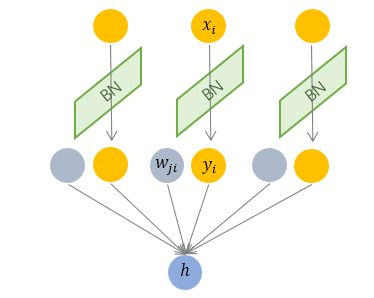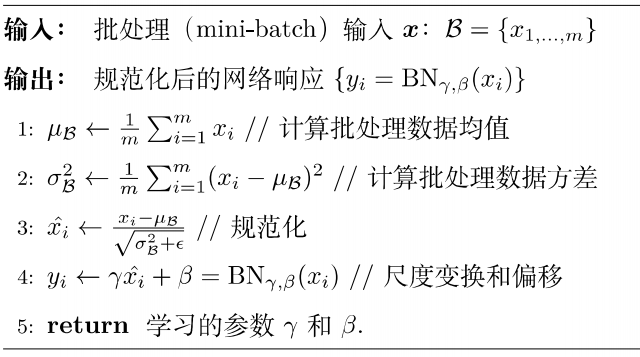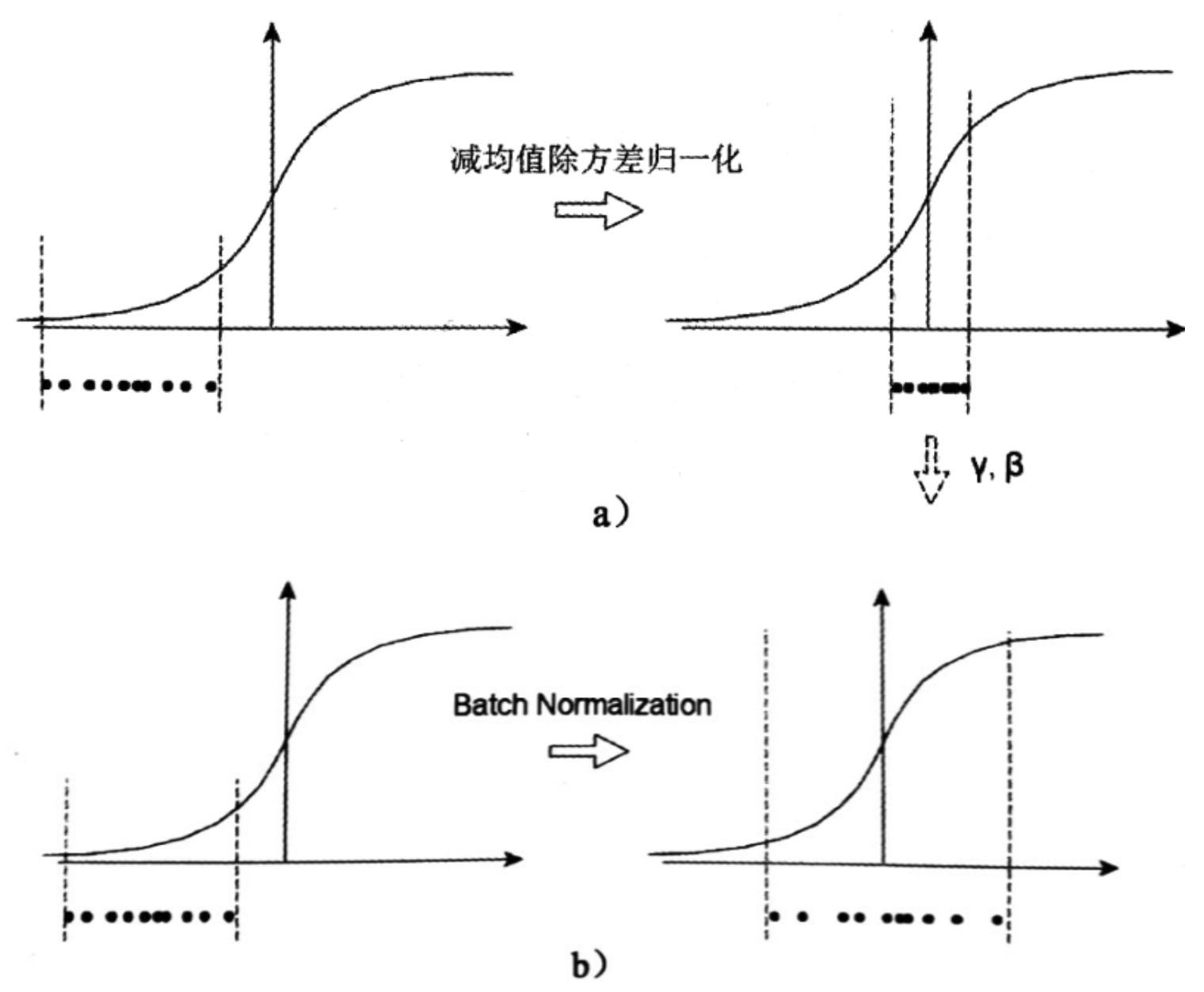## Normalization

### 独立同分布与白化

1. 去除特征之间的相关性 $$\longrightarrow$$ 独立
2. 使得所有特征具有相同的均值和方差 $$\longrightarrow$$ 同分布

### 深度学习中的 Internal Covariate Shift

Google 将这一现象总结为 Internal Covariate Shift，简称 ICS.

$$P_{s}(Y | X=x)=P_{t}(Y | X=x)$$

$$P_{s}(X) \neq P_{t}(X)$$

### ICS 会导致什么问题？

1. 上层参数需要不断适应新的输入数据分布，降低学习速度。

2. 下层输入的变化可能趋向于变大或者变小，导致上层落入饱和区，使得学习过早停止。

3. 每层的更新都会影响到其它层，因此每层的参数更新策略需要尽可能的谨慎。

## Normalization 的通用框架与基本思想

BN的基本思想其实相当直观：

$$h=f\left(\mathbf{g} \cdot \frac{\mathbf{x}-\mu}{\sigma}+\mathbf{b}\right)$$

1. $$\mu$$ 是平移参数（shift parameter）， $$\sigma$$ 是缩放参数（scale parameter）。通过这两个参数进行 shift 和 scale 变换： $$\hat{\mathbf{x}}=\frac{\mathbf{x}-\mu}{\sigma}$$ 得到的数据符合均值为 0、方差为 1 的标准分布。

2. $$\mathbf{b}$$ 是再平移参数（re-shift parameter），$$\mathbf{g}$$ 是再缩放参数（re-scale parameter）。将 上一步得到的 $$\hat{\mathbf{x}}$$ 进一步变换为：

$$\mathbf{y}=\mathbf{g} \cdot \hat{\mathbf{x}}+\mathbf{b}$$

## 主流 Normalization 方法梳理

### Batch Normalization 纵向规范化$$\mu_{i}=\frac{1}{M} \sum x_{i}, \quad \sigma_{i}=\sqrt{\frac{1}{M} \sum\left(x_{i}-\mu_{i}\right)^{2}+\epsilon}$$